# JP5317812B2 - Electric motor control device - Google Patents

## Info

Publication number
JP5317812B2
JP5317812B2 JP2009104925A JP2009104925A JP5317812B2 JP 5317812 B2 JP5317812 B2 JP 5317812B2 JP 2009104925 A JP2009104925 A JP 2009104925A JP 2009104925 A JP2009104925 A JP 2009104925A JP 5317812 B2 JP5317812 B2 JP 5317812B2
Authority
JP
Japan
Prior art keywords
phase
control device
state
electric motor
output voltage
Prior art date
Legal status (The legal status is an assumption and is not a legal conclusion. Google has not performed a legal analysis and makes no representation as to the accuracy of the status listed.)
Active
Application number
JP2009104925A
Other languages
Japanese (ja)
Other versions
JP2010259180A (en
Inventor

Original Assignee

Priority date (The priority date is an assumption and is not a legal conclusion. Google has not performed a legal analysis and makes no representation as to the accuracy of the date listed.)
Filing date
Publication date
Application filed by 本田技研工業株式会社 filed Critical 本田技研工業株式会社
Priority to JP2009104925A priority Critical patent/JP5317812B2/en
Publication of JP2010259180A publication Critical patent/JP2010259180A/en
Application granted granted Critical
Publication of JP5317812B2 publication Critical patent/JP5317812B2/en
Application status is Active legal-status Critical
Anticipated expiration legal-status Critical

## Images

•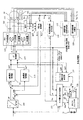•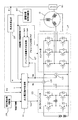•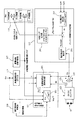•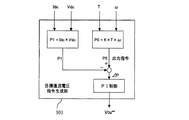••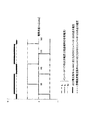•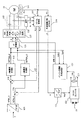•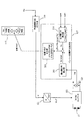•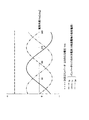•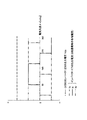•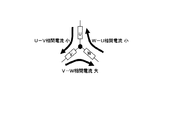•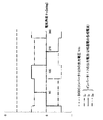## Description

The present invention relates to a control device for an electric motor having a rotor and a stator that rotates a rotor by generating a rotating magnetic field by an applied voltage.

FIG. 7 is a block diagram showing an electric motor control apparatus as a related technique of the present invention. 7 includes a resolver 101, a current sensor 103, a bandpass filter (BPF) 105, a three-phase-dp conversion unit 107, a current command calculation unit 109, and a d-axis current control unit. 111, q-axis current control unit 113, rθ conversion unit 115, inverter (INV) 117, angular velocity calculation unit 119, DC voltage command generation unit 121, DCDC converter 123, output voltage detection unit 125, And an inverter calculation method determination unit 127. Electric power is supplied from the battery 15 to the electric motor 10 shown in FIG. 7 via the control device. The electric motor 10 is, for example, a three-phase brushless DC motor that includes a rotor having permanent magnets and a stator that rotates a rotor by generating a rotating magnetic field using two-phase or three-phase applied voltages.

The resolver 101 detects the mechanical angle of the rotor of the electric motor 10 and outputs an electrical angle θm corresponding to the detected mechanical angle. The electrical angle θm output from the resolver 101 is sent to the three-phase-dp converter 107 and the angular velocity calculator 119. The current sensor 103 detects each phase current of the three-phase current supplied from the inverter 117 and supplied to the stator of the electric motor 10.

The BPF 105 removes unnecessary components of each current detection signal indicating the three-phase alternating currents Iu, Iv, Iw detected by the current sensor 103. The three-phase-dp conversion unit 107 performs three-phase-dq conversion based on the current detection signal from which unnecessary components have been removed by the BPF 105 and the electrical angle θm of the rotor detected by the resolver 101, and the d-axis current Detection value Id_s and q-axis current detection value Iq_s are calculated.

The current command calculator 109 is a current (hereinafter referred to as “d-axis current”) that flows through a d-axis side stator (hereinafter referred to as “d-axis stator”) based on a torque command value T input from the outside. Command value Id * and a command value Iq * of a current (hereinafter referred to as “q-axis current”) flowing through a q-axis side stator (hereinafter referred to as “q-axis stator”). The d-axis current command value Id * is input to the d-axis current control unit 111. Further, the q-axis current command value Iq * is input to the q-axis current control unit 113. The d axis is a field axis, and the q axis is a torque axis.

The d-axis current control unit 111 has a command value Vd for the voltage across the terminals of the d-axis stator (hereinafter referred to as “d-axis voltage”) so that the deviation ΔId between the command value Id * of the d-axis current and the detected value Id_s decreases. Determine **. The q-axis current control unit 113 controls the command value Vq of the inter-terminal voltage of the q-axis stator (hereinafter referred to as “q-axis voltage”) so that the deviation ΔIq between the q-axis current command value Iq * and the detected value Iq_s decreases. Determine **. The d-axis voltage command value Vd ** and the q-axis voltage command value Vq ** are input to the rθ conversion unit 115 and the inverter calculation method determination unit 127.

The rθ conversion unit 115 converts the command value Vd ** of the d-axis voltage and the command value Vq ** of the q-axis voltage into components of the voltage level V1 and the angle θ.

The inverter 117 converts the DC voltage from the battery 15 via the DCDC converter 123 into a three-phase (U, V, W) AC voltage based on the voltage level V1 and the angle θ component input from the rθ converter 115. Convert. The inverter 117 is a rectangular wave inverter, and performs either PWM (Pulse Width Modulation) control or one-pulse (1PLS) control according to the switching flag input from the inverter calculation method determination unit 127. Note that the PWM control can control the output voltage of the inverter 117 with higher accuracy as the switching frequency is higher. On the other hand, 1PLS control has a small switching loss because the switching frequency is low. Note that, during the 1PLS control, the inverter 117 performs either two-phase energization or three-phase energization for the electric motor 10.

The angular velocity calculation unit 119 calculates the angular velocity ω of the rotor of the electric motor 10 by time differentiation of the electrical angle θm output from the resolver 101. The angular velocity ω calculated by the angular velocity calculation unit 119 is input to the DC voltage command generation unit 121.

The DC voltage command generation unit 121 refers to a table in which the angular velocity ω and the output voltage command Vcu * correspond, and outputs a constant DC voltage corresponding to the angular velocity ω input from the angular velocity calculation unit 119. An output voltage command Vcu * is generated. The output voltage command Vcu * is input to the DCDC converter 123. The DCDC converter 123 boosts or lowers the direct current output voltage of the battery 15 while maintaining the direct current. The output voltage detector 125 detects the output voltage Vdc of the DCDC converter 123.

The inverter calculation method determination unit 127 includes the output voltage Vdc of the DCDC converter 123, the command value Vd ** of the d-axis voltage output from the d-axis current control unit 111, and the q-axis output from the q-axis current control unit 113. Based on the voltage command value Vq **, the switching flag to be input to the inverter 117 is determined.

FIG. 8 is a block diagram showing the internal configuration of the inverter calculation method determination unit 127 and the relationship with the components related thereto. As illustrated in FIG. 8, the inverter calculation method determination unit 127 includes a maximum voltage circle calculation unit 201, an output voltage circle calculation unit 203, and a switching flag output unit 205. The maximum voltage circle calculation unit 201 derives a value (Vdc / √6) Vp_target obtained by dividing the output voltage Vdc of the DCDC converter 123 by √6. This value Vp_target is the maximum value of the phase voltage that can be applied to the electric motor 10, that is, the phase voltage value that is applied to the electric motor 10 with the duty ratio in the inverter 117 being 100%.

The output voltage circle calculation unit 203 derives the calculation result of √ (Vd ** 2 + Vq ** 2 ) as a combined vector voltage Vp. The switching flag output unit 205 outputs a switching flag corresponding to the difference ΔVp (= Vp_target−Vp) between the value Vp_target derived by the maximum voltage circle calculation unit 201 and the combined vector voltage Vp derived by the output voltage circle calculation unit 203. To do. The switching flag output unit 205 outputs a switching flag indicating PWM control when the difference ΔVp is greater than 0 (ΔVp> 0), and outputs a flag indicating 1PLS control when the difference ΔVp is 0 or less (ΔVp ≦ 0). To do.

JP 2005-51894 A

In the control device for the electric motor 10 described above, the DCDC converter 123 is controlled to output a constant DC voltage corresponding to the angular velocity ω of the rotor of the electric motor 10. The inverter 117 performs PWM control when the difference ΔVp (= Vp_target−Vp) derived by the inverter calculation method determination unit 127 is greater than 0 (ΔVp> 0), and the difference ΔVp is 0 or less (ΔVp ≦ 0). Sometimes 1PLS control is performed.

As described above, the inverter 117 during 1PLS control performs either two-phase energization or three-phase energization for the electric motor 10. The stator of the electric motor 10 is provided with windings to which a phase current is supplied, and the windings of each phase are respectively star-connected (Y-connected). FIG. 9 is a graph showing the output voltage Vdc of the DCDC converter 123 and each phase current that the inverter 117 supplies to the electric motor 10 during three-phase energization. FIG. 10 is a graph showing the output voltage Vdc of the DCDC converter 123 and each phase current that the inverter 117 supplies to the electric motor 10 during two-phase energization.

Depending on the temperature difference of the portion (coil) corresponding to each phase of the stator of the electric motor 10, the inductance difference of the winding, etc., there may be a deviation between the phase currents. As the temperature of the stator coil increases, the resistance component of the coil increases. For example, in a state where the temperature of the U-phase coil is particularly higher than the temperatures of the other two-phase (V-phase and W-phase) coils (one-phase temperature concentration state), as shown in FIG. The current and the W-U phase current are smaller than the V-W phase current.

FIG. 12 shows the output voltage Vdc of the DCDC converter 123 and the electric motor 10 when the inverter 117 performs two-phase energization in a state where the temperature of the U-phase coil is higher than the temperatures of the other two-phase coils. It is a graph which shows each phase current. Note that the output voltage of the DCDC converter 123 is constant. As shown in FIG. 12, if the phase current supplied from the inverter 117 to the electric motor 10 is biased, the torque of the electric motor 10 may fluctuate and noise and vibration may be generated. Further, the current concentrates on some switching elements in the inverter 117, and the switching elements are more likely to be damaged due to excessive temperature rise. Thus, the deviation between the phase currents causes a decrease in the operating efficiency of the electric motor 10.

An object of the present invention is to provide a control device for an electric motor capable of operating the electric motor while maintaining the operation efficiency even under a condition in which bias may occur between phase currents.

In order to solve the above-described problems and achieve the object, an electric motor control device according to claim 1 includes a rotor, a stator that generates a rotating magnetic field by an applied voltage, and rotates the rotor. And an inverter that drives the motor by supplying a phase current to the stator of the motor (for example, an inverter in the embodiment) 117), a voltage converter (for example, DCDC converter 123 in the embodiment) that boosts or steps down an output voltage of a DC power source (for example, the battery 15 in the embodiment) and applies the voltage to the inverter, and the voltage An output voltage command generation unit (for example, output voltage command generation unit 151 in the embodiment) that generates an output voltage command for instructing an output voltage of the conversion unit, and the inverter during rectangular wave control An energization mode switching unit (for example, an energization mode switching unit 157 in the embodiment) that switches an energization mode performed by the motor and an electrical angle acquisition unit (for example, in the embodiment) that acquires an electrical angle of the rotor of the electric motor And a state detection unit that detects a first state in which a bias occurs between the phase currents supplied to the electric motor by the inverter or a second state that satisfies a condition in which the bias occurs between the phase currents. (For example, when the state detection unit detects the first state or the second state, the energization mode switching unit is configured to supply the energization mode). And the output voltage command generator is instructed to correct the bias between the phase currents in synchronization with a change in the electrical angle of the rotor acquired by the electrical angle acquisition unit. Output voltage It is characterized by generating a decree.

Furthermore, in the motor control device according to the second aspect of the present invention, an electric motor (for example, in the embodiment) having a rotor and a stator that generates a rotating magnetic field by an applied voltage and rotates the rotor. A controller for the electric motor 10), which supplies a phase current to the stator of the electric motor to drive the electric motor (for example, the inverter 117 in the embodiment), and a DC power source (for example, the embodiment) The voltage converter (for example, the DCDC converter 123 in the embodiment) that boosts or steps down the output voltage of the battery 15) and applies it to the inverter, and the output voltage for indicating the output voltage of the voltage converter An output voltage command generation unit (for example, an output voltage command generation unit 151 in the embodiment) that generates a command, and an energization that switches an energization mode performed by the inverter during rectangular wave control The state switching unit (for example, the energization mode switching unit 157 in the embodiment) and the first state in which the bias is generated between the phase currents supplied to the electric motor by the inverter, or the bias is generated between the phase currents. A state detection unit (for example, an energization mode switching unit 157 in the embodiment) that detects a second state that satisfies the condition, and the state detection unit detects the first state or the second state Then, the energization mode switching unit switches the energization mode to two-phase energization, and the output voltage command generation unit synchronizes with the change in the phase current supplied to the electric motor. The output voltage command for instructing to correct the bias is generated.

Furthermore, in the motor control device according to claim 3, the state detection unit includes a phase current detection unit that detects the phase current, and any one of the phase currents detected by the phase current detection unit. When the difference between the integrated current value of one phase current and the integrated current value of another phase current is equal to or greater than a threshold value, the control device detects that the first state is present.

Furthermore, in the motor control device according to claim 4, the state detection unit includes a temperature detection unit that detects a temperature of a portion corresponding to each phase of the stator, and the temperature detection unit detects the temperature. When the difference between any one of the temperatures and the other temperature is equal to or greater than a threshold value, the control device detects the second state.

Furthermore, in the motor control device according to the fifth aspect of the present invention, the state detection unit includes a temperature detection unit that detects a temperature of a portion corresponding to each phase of the stator, and the temperature detection unit detects the temperature detection unit. When any one of the temperatures is equal to or higher than a threshold value, the control device detects the second state.

Furthermore, in the motor control device according to claim 6, the state detection unit includes an inductance deriving unit that derives an inductance of each phase coil constituting the stator, and each of the inductance deriving units derived by the inductance deriving unit. When the difference between any one of the inductances of the phase coils and the other inductance is equal to or greater than a threshold value, the control device detects the second state.

Furthermore, in the motor control device according to claim 7, the state detection unit includes an inductance deriving unit that derives an inductance of each phase coil constituting the stator, and each of the inductance deriving units derived by the inductance deriving unit. When any one of the phase coil inductances is equal to or greater than a threshold value, the control device detects the second state.

Furthermore, in the motor control apparatus according to an eighth aspect of the present invention, the state detection unit includes a resistance component deriving unit that derives a resistance component of a winding of each phase coil constituting the stator, and the resistance component When the difference between any one of the resistance components of the windings of each phase coil derived by the deriving unit and the other resistance component is equal to or greater than a threshold value, the control device detects that the second state is detected. It is characterized by doing.

Furthermore, in the motor control device according to claim 9, the state detection unit includes a resistance component deriving unit for deriving a resistance component of a winding of each phase coil constituting the stator, and the resistance component The control device detects the second state when any one of the resistance components of the winding of each phase coil derived by the deriving unit is greater than or equal to a threshold value.

According to the motor control device of the first to ninth aspects of the present invention, it is possible to operate the motor while maintaining the operation efficiency even under the condition that the bias may occur between the phase currents.

The block diagram which shows the control apparatus of the electric motor of one Embodiment 1 is a block diagram showing a part of the control device for the electric motor 10 shown in FIG. 1 and each circuit of a DCDC converter and an inverter 117. As a part of the control device of the electric motor 10 shown in FIG. 1, a block diagram showing the internal configurations of the output voltage command generation unit 151 and the inverter calculation method determination unit 127 and the relationship with the components related thereto. The block diagram which shows the internal structure of the target DC voltage command generation part 301 which the output voltage command generation part 151 has When the inverter 117 conducts two-phase energization in a state where the temperature of the U-phase coil is higher than the temperatures of the other two-phase coils, the DCDC converter 123 performs a boost operation in accordance with the target DC voltage command Vcu **. Graph showing each phase current supplied to the electric motor 10 by the inverter 117 and the voltage output by the DCDC converter 123 according to the target DC voltage command Vcu ** When the inverter 117 performs two-phase energization while the temperature of the U-phase coil is higher than the temperatures of the other two-phase coils, the DCDC converter 123 performs a boost operation according to the output voltage command Vcu ***. Is a graph showing each phase current supplied to the electric motor 10 and each voltage output by the DCDC converter 123 according to the target DC voltage command Vcu ** and the output voltage command Vcu ***. The block diagram which shows the control apparatus of the electric motor as related technology of this invention The block diagram which shows the relationship between the internal structure of the inverter calculation system determination part 127 shown in FIG. 7, and the component related to this. The graph which shows the output voltage Vdc of the DCDC converter 123, and each phase voltage which the inverter 117 applies to the electric motor 10 at the time of three-phase electricity supply The graph which shows the output voltage Vdc of the DCDC converter 123, and each phase voltage which the inverter 117 applies to the electric motor 10 at the time of two-phase electricity supply The figure which shows the electric current between each phase when the inverter 117 performs two-phase electricity supply in the state where the temperature of the coil of a U phase is higher than each temperature of another two-phase coil The output voltage Vdc of the DCDC converter 123 and each phase voltage applied to the motor 10 when the inverter 117 conducts two-phase energization in a state where the temperature of the U-phase coil is higher than each temperature of the other two-phase coils. Graph showing

Hereinafter, embodiments of the present invention will be described with reference to the drawings.

FIG. 1 is a block diagram illustrating an electric motor control apparatus according to an embodiment. The control device for the electric motor 10 shown in FIG. 1 includes a resolver 101, a current sensor 103, a band-pass filter (BPF) 105, a three-phase-dp conversion unit 107, as in the electric motor control device shown in FIG. , Current command calculation unit 109, d-axis current control unit 111, q-axis current control unit 113, rθ conversion unit 115, inverter (INV) 117, angular velocity calculation unit 119, DCDC converter 123, and output voltage A detection unit 125 and an inverter calculation method determination unit 127; an output current detection unit 153; a temperature sensor 155; an energization mode switching determination unit 157; and a resistance increase phase determination unit 159; Instead, an output voltage command generation unit 151 is provided. In FIG. 1, the same reference numerals are assigned to components common to FIG. 7.

FIG. 2 is a block diagram showing a part of the control device of the electric motor 10 shown in FIG. 1 and each circuit of the DCDC converter and the inverter 117. As shown in FIG. 2, the DCDC converter 123 used in this embodiment is a step-up / down converter. The output current detector 153 shown in FIGS. 1 and 2 detects the output current Idc of the DCDC converter 123. The temperature sensor 155 detects each temperature (hereinafter referred to as “coil temperature”) of a portion (coil) corresponding to each phase of the stator of the electric motor 10.

A current detection signal indicating the three-phase alternating currents Iu, Iv, Iw and a temperature detection signal indicating the coil temperature of each phase detected by the temperature sensor 155 are input to the energization mode switching determination unit 157. Based on the three-phase AC currents Iu, Iv, and Iw indicated by the phase current detection signal, the energization mode switching determination unit 157 is in a state in which the phase current is biased depending on the phase when the inverter 117 performs two-phase energization during 1PLS control. Determine whether or not. Note that the energization mode switching determination unit 157 determines that the difference between the integrated current value of any one of the three-phase alternating currents Iu, Iv, and Iw and the integrated current value of the other two phase currents is a threshold value. At this time, it is determined that the phase current is biased.

In addition, based on the temperature detection signal indicating the coil temperature of each phase, the energization mode switching determination unit 157 has a high possibility that the phase current is biased depending on the phase when the inverter 117 during 1PLS control performs two-phase energization Determine whether or not. In addition, when the difference between any one coil temperature and the other two coil temperatures is equal to or greater than a threshold value among the coil temperatures of each phase, the energization mode switching determination unit 157 is likely to cause the phase current to be biased. Judged as a state. The energization mode switching determination unit 157 determines that the phase current is highly likely to be biased when any one of the coil temperatures of each phase is equal to or higher than a threshold value. For example, in the one-phase temperature concentration state where the coil temperature of the U phase is particularly higher than the coil temperatures of the other two phases (V phase and W phase), as shown in FIG. The U-phase current is likely to be smaller than the V-W phase current.

The inverter 117 of the present embodiment performs three-phase energization or two-phase energization with dead time for the motor 10 during 1PLS control. When it is determined that the phase current is in a biased state or a state in which the phase current is likely to be biased, the energization mode switching determination unit 157 switches the energization mode performed by the inverter 117 during 1PLS control to two-phase energization. At this time, the energization mode switching determination unit 157 outputs a switching flag indicating two-phase energization. Further, when the energization mode switching determination unit 157 determines that neither the state in which the phase current is biased or the state in which the phase current is likely to be biased is high, the energization mode performed by the inverter 117 during the 1PLS control is switched to three-phase energization . At this time, the energization mode switching determination unit 157 outputs a switching flag indicating three-phase energization. The two-phase energization / 3-phase energization switch flag output from the energization mode switching determination unit 157 is input to the inverter 117 and the output voltage command generation unit 151.

The resistance increase phase determination unit 159 receives a phase current detection signal indicating the three-phase AC currents Iu, Iv, and Iw and a temperature detection signal indicating the coil temperature of each phase detected by the temperature sensor 155. Based on the phase current detection signal or the temperature detection signal, the resistance increase phase determination unit 159 determines the difference between the resistance components between the portions corresponding to the respective phases of the stator of the electric motor 10 and (Hereinafter referred to as “resistance increasing phase”). The difference between the resistance increasing phase and the resistance component (hereinafter referred to as “phase resistance information”) determined by the resistance increasing phase determining unit 159 is input to the output voltage command generating unit 151.

FIG. 3 shows each internal configuration of the output voltage command generation unit 151 and the inverter calculation method determination unit 127 as a part of the control device of the electric motor 10 shown in FIG. 1 and the relationship with the related components. It is a block diagram. As shown in FIG. 3, the inverter calculation method determination unit 127 includes a maximum voltage circle calculation unit 201, an output voltage circle calculation unit 203, and a switching flag output unit 205. The maximum voltage circle calculation unit 201 derives a value (Vdc / √6) Vp_target obtained by dividing the output voltage Vdc of the DCDC converter 123 by √6. This value Vp_target is the maximum value of the phase voltage that can be applied to the electric motor 10, that is, the phase voltage value that is applied to the electric motor 10 with the duty ratio in the inverter 117 being 100%.

The output voltage circle calculation unit 203 derives the calculation result of √ (Vd ** 2 + Vq ** 2 ) as a combined vector voltage Vp. The switching flag output unit 205 performs PWM / 1PLS switching according to the difference ΔVp (= Vp_target−Vp) between the value Vp_target derived by the maximum voltage circle calculation unit 201 and the combined vector voltage Vp derived by the output voltage circle calculation unit 203. Output a flag. The switching flag output unit 205 outputs a switching flag indicating PWM control when the difference ΔVp is greater than 0 (ΔVp> 0), and outputs a flag indicating 1PLS control when the difference ΔVp is 0 or less (ΔVp ≦ 0). To do. The PWM / 1PLS switching flag output from the switching flag output unit 205 is input to the inverter 117.

As shown in FIG. 3, the output voltage command generation unit 151 includes a target DC voltage command generation unit 301 and a voltage command output unit 303. As shown in FIGS. 1 to 3, the output voltage command generation unit 151 outputs the torque command value T input from the outside, the angular velocity ω calculated by the angular velocity calculation unit 119, and the resolver 101. The electrical angle θm, the output voltage Vdc of the DCDC converter 123 detected by the output voltage detection unit 125, the output current Idc of the DCDC converter 123 detected by the output current detection unit 127, and the two phases output by the energization mode switching determination unit 157 The energization / 3-phase energization switching flag and the phase resistance information output by the resistance increase phase determination unit 159 are input.

FIG. 4 is a block diagram illustrating an internal configuration of the target DC voltage command generation unit 301 included in the output voltage command generation unit 151. As shown in FIG. 4, the target DC voltage command generation unit 301 multiplies the coefficient K, the torque command value T, and the angular velocity ω to derive the required output power P0. The target DC voltage command generation unit 301 multiplies the output current Idc of the DCDC converter 123 by the output voltage Vdc to derive the actual output power P1. The target DC voltage command generation unit 301 generates a target DC voltage command Vcu ** as a control amount of PI control according to the difference ΔP (= P0−P1) between the requested output power P0 and the actual output power P1. The target DC voltage command Vcu ** is input to the voltage command output unit 303.

The voltage command output unit 303 outputs the electric power of the rotor of the electric motor 10 based on the phase resistance information output by the resistance increase phase determination unit 159 when the energization mode switching determination unit 157 outputs a flag indicating two-phase energization. An output voltage command Vcu *** is output to instruct the DCDC converter 123 to boost the voltage at a transformation rate synchronized with the angle θm. On the other hand, when the energization mode switching determination unit 157 outputs a flag indicating three-phase energization, the voltage command output unit 303 outputs the target DC voltage command Vcu ** generated by the target DC voltage command generation unit 301 as the output voltage. Output as command Vcu ***. The output voltage command 7Vcu *** output from the voltage command output unit 303 is input to the DCDC converter 123.

FIG. 5 shows that when the inverter 117 conducts two-phase energization in a state where the temperature of the U-phase coil is higher than the temperatures of the other two-phase coils, the DCDC converter 123 responds to the target DC voltage command Vcu **. It is a graph which shows the voltage which the DCDC converter 123 outputs according to each phase current which the inverter 117 supplies to the electric motor 10, and target DC voltage command Vcu ** when performing a pressure | voltage rise operation. Note that the output voltage of the DCDC converter 123 corresponding to the target DC voltage command Vcu ** is constant.

When the U-phase coil temperature is higher than the other two-phase coil temperatures, the resistance component of the winding provided in the U-phase coil is larger than the resistance component of the winding of the other-phase coil. At this time, as shown in FIG. 5, there is a high possibility that deviation occurs between the phase currents supplied to the electric motor 10 by the inverter 117, or such deviation occurs. The voltage command output unit 303 adjusts the step-up rate according to the electrical angle θm based on the phase resistance information so as to equalize the three-phase current values output from the inverter 117 by the output voltage command Vcu ***.

FIG. 6 shows that when the inverter 117 conducts two-phase energization in a state where the temperature of the U-phase coil is higher than the temperatures of the other two-phase coils, the DCDC converter 123 responds to the output voltage command Vcu ***. 4 is a graph showing each phase current supplied to the electric motor 10 when the boosting operation is performed, and each voltage output by the DCDC converter 123 according to the target DC voltage command Vcu ** and the output voltage command Vcu ***. . As shown in FIG. 6, the inverter 117 is compared with the output voltage of the DCDC converter 123 according to the target DC voltage command Vcu ** by the output voltage of the DCDC converter 123 according to the output voltage command Vcu ***. The three-phase current values output from are equal regardless of the electrical angle θm.

As described above, according to the motor control device of the present embodiment, when the inverter 117 during the 1PLS control is biased between the phase currents when the two-phase energization is performed, or there is a possibility thereof Based on the phase resistance information, the transformation rate of the DCDC converter 123 is changed according to the electrical angle θm of the rotor of the electric motor 10. As a result, since the current values of the three phases output from the inverter 117 are equal, the torque of the electric motor 10 does not vary due to the deviation between the phase currents, and the switching element of the inverter 117 is not damaged due to excessive temperature rise. . Therefore, the electric motor 10 can be driven with high operation efficiency.

In the above embodiment, the output voltage command generation unit 121 outputs the output voltage command Vcu *** in synchronization with the electrical angle θm of the rotor of the electric motor 10, but the electric motor 10 detected by the current sensor 103 is output. The output voltage command Vcu *** may be output in synchronization with the three-phase current supplied to the stator. In addition, although the DCDC converter 123 of this embodiment is a step-up / step-down converter, it may be a step-up converter or a step-down converter.

In the above-described embodiment, the energization mode switching unit 157 may cause the bias between the phase currents or the phase current based on the three-phase AC currents Iu, Iv, Iw or the coil temperature of each phase. It is judged whether or not the state is high. As another embodiment, the energization mode switching unit 157 may cause a bias between the phase currents or a state in which the bias between the phase currents is generated based on the inductance of each phase coil constituting the stator of the electric motor 10. It may be determined whether or not the state is high. If the inductance increases, the impedance also increases, so there is a high possibility that a bias will occur between the phase currents. Further, the energization mode switching unit 157 may determine that the phase current is likely to be biased when any one of the inductances of the respective phase coils is equal to or greater than a threshold value.

The inductance of each phase coil is calculated for each phase by the energization mode switching unit 157 using the following equation (1). The resistance R is the resistance of the q-axis armature and the d-axis armature, and is a preset fixed value. The d-axis component inductance Ld is constant with respect to the magnitude of the phase current.

Further, as another embodiment, the energization mode switching unit 157 has a high possibility of occurrence of bias between phase currents or occurrence of bias between phase currents based on the resistance component of the winding of each phase coil. You may judge whether it is in a state. The energization mode switching unit 157 may determine that the phase current is likely to be biased when any one of the resistance components of the windings of each phase coil is equal to or greater than the threshold value. . The resistance component of the winding of each phase coil is calculated for each phase by the energization mode switching unit 157 using simultaneous equations of the following equations (2) and (3). Note that Ke is an induced voltage constant.

DESCRIPTION OF SYMBOLS 10 Electric motor 15 Capacitor 101 Resolver 103 Current sensor 105 Band pass filter (BPF)
107 three-phase-dp conversion unit 109 current command calculation unit 111 d-axis current control unit 113 q-axis current control unit 115 rθ conversion unit 117 inverter 119 angular velocity calculation unit 123 DCDC converter 125 output voltage detection unit 127 inverter calculation method determination unit 151 output Voltage command generation unit 153 Output current detection unit 155 Temperature sensor 157 Energization mode switching determination unit 159 Resistance increase phase determination unit 201 Maximum voltage circle calculation unit 203 Output voltage circle calculation unit 205 Switching flag output unit 301 Target DC voltage command generation unit 303 Voltage Command output section

## Claims (9)

1. A control device for an electric motor having a rotor, and a stator that rotates a rotor by generating a rotating magnetic field by an applied voltage,
An inverter that drives the electric motor by supplying a phase current to the stator of the electric motor;
A voltage converter for stepping up or stepping down the output voltage of the DC power source and applying it to the inverter;
An output voltage command generator for generating an output voltage command for instructing an output voltage of the voltage converter;
An energization mode switching unit that switches an energization mode performed by the inverter during rectangular wave control;
An electrical angle acquisition unit for acquiring an electrical angle of the rotor of the electric motor;
A first state in which a bias has occurred between the phase currents supplied to the electric motor by the inverter, or a state detection unit that detects a second state that satisfies a condition in which the bias occurs between the phase currents,
When the state detection unit detects the first state or the second state, the energization mode switching unit switches the energization mode to two-phase energization, and the output voltage command generation unit The motor control device according to claim 1, wherein the output voltage command is generated to instruct to correct the bias between the phase currents in synchronization with a change in the electrical angle of the rotor acquired by the angle acquisition unit.
2. A control device for an electric motor having a rotor, and a stator that rotates a rotor by generating a rotating magnetic field by an applied voltage,
An inverter that drives the electric motor by supplying a phase current to the stator of the electric motor;
A voltage converter for stepping up or stepping down the output voltage of the DC power source and applying it to the inverter;
An output voltage command generator for generating an output voltage command for instructing an output voltage of the voltage converter;
An energization mode switching unit that switches an energization mode performed by the inverter during rectangular wave control;
A first state in which a bias has occurred between the phase currents supplied to the electric motor by the inverter, or a state detection unit that detects a second state that satisfies a condition in which the bias occurs between the phase currents,
When the state detection unit detects the first state or the second state, the energization mode switching unit switches the energization mode to two-phase energization, and the output voltage command generation unit includes the electric motor A control apparatus for an electric motor, wherein the output voltage command is generated to instruct to correct a deviation between the phase currents in synchronization with a change in the phase current supplied to the motor.
3. The motor control device according to claim 1 or 2,
The state detection unit includes a phase current detection unit that detects the phase current,
When the difference between the accumulated current value of any one of the phase currents detected by the phase current detection unit and the accumulated current value of the other phase currents is greater than or equal to a threshold value, the control device A control device for an electric motor characterized by detecting the state of the motor.
4. The motor control device according to claim 1 or 2,
The state detection unit includes a temperature detection unit that detects a temperature of a portion corresponding to each phase of the stator,
When the difference between any one of the temperatures detected by the temperature detection unit and the other temperature is equal to or greater than a threshold value, the control device detects the second state. Control device.
5. The motor control device according to claim 1 or 2,
The state detection unit includes a temperature detection unit that detects a temperature of a portion corresponding to each phase of the stator,
The motor control device, wherein when any one of the temperatures detected by the temperature detection unit is equal to or higher than a threshold value, the control device detects the second state.
6. The motor control device according to claim 1 or 2,
The state detection unit includes an inductance deriving unit for deriving the inductance of each phase coil constituting the stator,
When the difference between any one of the inductances of each phase coil derived by the inductance deriving unit and the other inductance is equal to or greater than a threshold value, the control device detects the second state. An electric motor control device.
7. The motor control device according to claim 1 or 2,
The state detection unit includes an inductance deriving unit for deriving the inductance of each phase coil constituting the stator,
The control device for an electric motor, wherein when any one of the inductances of the respective phase coils derived by the inductance deriving unit is equal to or greater than a threshold value, the control device detects the second state.
8. The motor control device according to claim 1 or 2,
The state detection unit includes a resistance component deriving unit for deriving a resistance component of a winding of each phase coil constituting the stator,
When the difference between any one of the resistance components of the coils of each phase coil derived by the resistance component deriving unit and the other resistance component is equal to or greater than a threshold value, the control device An electric motor control device that detects a state.
9. The motor control device according to claim 1 or 2,
The state detection unit includes a resistance component deriving unit for deriving a resistance component of a winding of each phase coil constituting the stator,
The control device detects the second state when any one of the resistance components of the winding of each phase coil derived by the resistance component deriving unit is greater than or equal to a threshold value. The motor control device.
JP2009104925A 2009-04-23 2009-04-23 Electric motor control device Active JP5317812B2 (en)

## Priority Applications (1)

Application Number Priority Date Filing Date Title
JP2009104925A JP5317812B2 (en) 2009-04-23 2009-04-23 Electric motor control device

## Applications Claiming Priority (1)

Application Number Priority Date Filing Date Title
JP2009104925A JP5317812B2 (en) 2009-04-23 2009-04-23 Electric motor control device

## Publications (2)

Publication Number Publication Date
JP2010259180A JP2010259180A (en) 2010-11-11
JP5317812B2 true JP5317812B2 (en) 2013-10-16

# Family

## Family Applications (1)

Application Number Title Priority Date Filing Date
JP2009104925A Active JP5317812B2 (en) 2009-04-23 2009-04-23 Electric motor control device

## Country Status (1)

JP (1) JP5317812B2 (en)

## Families Citing this family (1)

* Cited by examiner, † Cited by third party
Publication number Priority date Publication date Assignee Title
JP6070430B2 (en) * 2013-06-18 2017-02-01 株式会社明電舎 Two-stage change prevention method and apparatus for serial multiple inverter control device

## Family Cites Families (4)

* Cited by examiner, † Cited by third party
Publication number Priority date Publication date Assignee Title
JP2001037282A (en) * 1999-07-15 2001-02-09 Toyota Motor Corp Electric controller for multiphase ac motor
JP2005027391A (en) * 2003-06-30 2005-01-27 Mitsuba Corp Driver of brushless motor
JP4280573B2 (en) * 2003-07-31 2009-06-17 トヨタ自動車株式会社 Load drive device
JP5047582B2 (en) * 2006-10-18 2012-10-10 東芝キヤリア株式会社 Inverter device

## Also Published As

Publication number Publication date
JP2010259180A (en) 2010-11-11

## Similar Documents

Publication Publication Date Title
JP4582168B2 (en) Rotating machine control device and rotating machine control system
EP2161828B1 (en) Motor drive system and its control method
US8278855B2 (en) Controller of motor preventing an increase in inverter loss
JP3627683B2 (en) Motor control device
WO2010038727A1 (en) Ac electric motor controlling apparatus and controlling method
US9054613B2 (en) Motor drive apparatus and vehicle with the same mounted thereon
JP4022630B2 (en) Power conversion control device, power conversion control method, and program for power conversion control
DE112010000463T5 (en) Control device for an electric motor drive device
CN101803177B (en) Controller for electric motor
JP2004187407A (en) Motor control equipment
US8829829B2 (en) Apparatus for calculating rotational position of rotary machine
KR101109067B1 (en) Position sensorless control apparatus of permanent magnet motor
JP2011083068A (en) Device for controlling motor driver
JP4406552B2 (en) Electric motor control device
WO2010026699A1 (en) Power conversion device
CN101577522B (en) Apparatus for carrying out improved control of rotary machine
US20110279071A1 (en) Control device for ac motor
JP5471255B2 (en) Control device for motor drive device
JP5471259B2 (en) Control device
JP4433099B1 (en) Power converter
JP2006311770A (en) Controller of motor drive system
EP2219290B1 (en) Ac motor controller
JP2005204350A (en) Controller of rotary electric machine and power generation system
JP4819970B2 (en) Electric motor drive power converter
CN101160713A (en) Controller for ac motor

## Legal Events

Date Code Title Description
A621 Written request for application examination

Free format text: JAPANESE INTERMEDIATE CODE: A621

Effective date: 20111125

A521 Written amendment

Free format text: JAPANESE INTERMEDIATE CODE: A523

Effective date: 20120522

TRDD Decision of grant or rejection written
A01 Written decision to grant a patent or to grant a registration (utility model)

Free format text: JAPANESE INTERMEDIATE CODE: A01

Effective date: 20130611

A61 First payment of annual fees (during grant procedure)

Free format text: JAPANESE INTERMEDIATE CODE: A61

Effective date: 20130709

R150 Certificate of patent or registration of utility model

Free format text: JAPANESE INTERMEDIATE CODE: R150